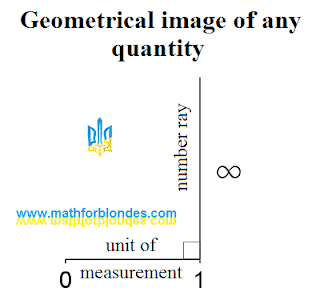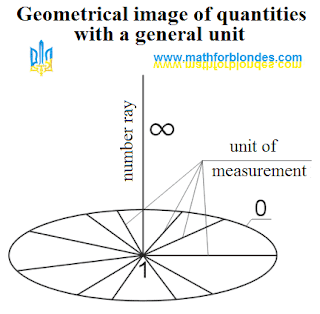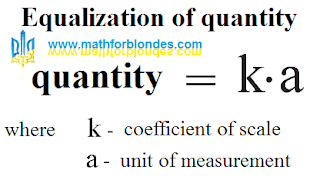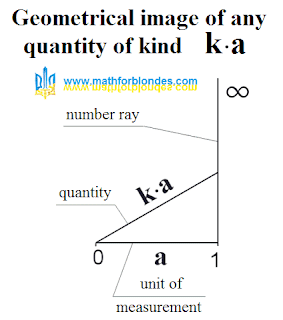## 10/19/2011

### Quantity as basis of mathematics

Co-operation of numbers and units of measurements takes place in a point "unit" and expressed by a mathematical action by an multiplication. Geometrically unit of measurement is perpendicular to the numerical ray. The result of multiplication of numbers on unit of measurement in future will be named "quantity". All quantities are identical mathematical characteristics initially.Geometrical image of any quantity

All units of measurements in the surrounding us world it is possible to depict two methods: with a general point "unit" and with a general point "zero". The method of image does not influence on properties of making elements.

If to take unit for a general point, then this will be a circumference with a numerical ray going out the center of circumference. The radiuses of circumference will be units of measurements.Geometrical image of quantities with a general unit

Approximately it is so possible to represent any universe with all present in her units of measurements. The image of all units of measurements as radiuses of circumference underlines a that circumstance, that all units of measurements are identical mathematical characteristics initially. (Expl for blondes: And you does not it seem to that this portrait of mathematics very reminds the ancient invention of man - wheel? Are you exactly sure that a wheel was invented exactly by a man? Maybe, did someone try to explain to the man, what mathematics, but he so nothing and did not understand? In memory of meeting with the unknown teachers of mathematics history left us only a wheel... Why did unknown teachers begin the story exactly with it? Because not knowing and not understanding such elementary things, understanding to the mathematician is practically impossible.That the previous generations of mathematicians were brilliantly demonstrated us.)Geometrical image of quantities with a general zero

Approximately it is so possible to represent the moment of appearance of universe, that it is accepted to name "Big Bang". In this case a numerical ray graphicly can be presented as a numerical cone.

In an algebraic kind any quantity can be presented by multiplying of coefficient of scale by unit of measurement. Numbers come forward as a coefficient of scale.Equalization of quantity

Geometrically any quantity that is the result of multiplication of coefficient of scale on unit of measurement, it is possible to present as a hypotenuse of rectangular triangle the cathetuses of that are unit of measurement and part of numerical ray.Geometrical image of any quantity of kind ka

Expl for blondes: Here now we got to one of types of mathematical corners we will consider and farther, what corner of scale and as there is a change of corner of scale. More interesting things on the page "New Math".